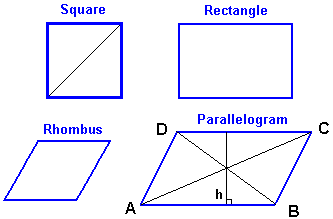## Parallelogram, Square, Rhombus and Rectangle Calculator

Click on the data you know:

Square - 1 Side Square - 1 Diagonal

Rectangle - 2 Sides

Rhombus - 1 Side and 1 Aangle

Parallelogram - 2 Sides and 1 Aangle

Significant Figures >>>

Lines AC and DB are called diagonals.

Line 'h' is perpendicular to lines AB & DC and is called the height or altitude.Parallelogram area = height × AB or DC

ALL PARALLELOGRAMS have the following properties:
1) BOTH pairs of opposite sides are equal.
2) BOTH pairs of opposite angles are equal.
∠ ADC = ∠ ABC and ∠ DAB = ∠ DCB
3) The diagonals bisect each other.
4) Each diagonal forms 2 congruent triangles.
Δ ADC = Δ ABC and Δ DAB = Δ DCB

Three special parallelograms have the above 4 properties and some additional ones.

square-all sides are equal
-all angles are equal
-both diagonals are equal
-diagonals meet at right angles

rectangle: -all angles are equal
-both diagonals are equal

rhombus: -all sides are equal
-diagonals meet at right angles

Thinkcalculator.com provides you helpful and handy calculator resources.